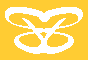Argument list
> Home
v User Guide
> Introduction
> Hello World
> Flow Control
v Function Calls
o Arguments
o Argument list
o Absolute arguments
o Results
o Pass by reference
> Authentication
> Database access
> Content Management
> Remote Calling
> Object-Oriented
> Other Features
> Reference
> Portal Object
> Development Guide

Shortcuts
sys Class
debug Class
Intrinsic Conversions
 >> User Guide >> Function Calls >> Argument list <=  =>      <  1  >
 Argument list
 A function can "discover" its argument list from the arg%function variable.  However, "function" is itself an argument, which contains the function name.  If you are not interested in the function name, you can ignore it (or clear it before you loop through the argument list using "foreach"): function sumup {    var result%function = 0;    clear arg%function:function;  // Clear the function name    foreach (arg%function) var result%function conv="+=:{foreach}";    display "{arg%function|list:(@key) + ()} = ";    display "{arg%function|list:(@value) + ()} = ";    display "{result%function}
\n"; }   sumup a=1 b=2 c=3;  // Output: a + b + c = 1 + 2 + 3 = 6 display "The sum is {result%sumup}
\n";  // Output: The sum is 6   Besides the special arg%function:function argument, if the function is called as a method of an object, you will have arg%function:this (the object name in the parent scope) and arg%function:thisfunction (the function name in the parent scope, which can be different from the one in the declaration line, denoted by arg%function:function.) Every BEE Variable is an array.  An argument is no different.  In the example of the function "sumup", you can pass an array of numbers instead of separate numbers. function sumup {    var result%function = 0;    foreach (arg%numbers) var result%function conv="+=:{foreach}";    display "{arg%numbers|list:(@key) + ()} = ";    display "{arg%numbers|list:(@value) + ()} = ";    display "{result%function}
\n"; }   sumup numbers="(array)a=>1,b=>2,c=>3";  // Output: a + b + c = 1 + 2 + 3 = 6 display "The sum is {result%sumup}
\n";  // Output: The sum is 6   You may use a variable in the calling line: var myArr = "(array)a=>1,b=>2,c=>3"; sumup numbers="(var)myArr";  // Output: a + b + c = 1 + 2 + 3 = 6 Previous Page       Next Page

Accsoft Computer Technology Pty Ltd     ABN: 98 065 617 549
PO Box 892, Epping NSW 1710         Level 1, Epping Office Park, 242 Beecroft Rd, Epping NSW 2121, Australia
Tel: Sydney - (02)98691668     National - 1300-881668         Fax: (02)98691866